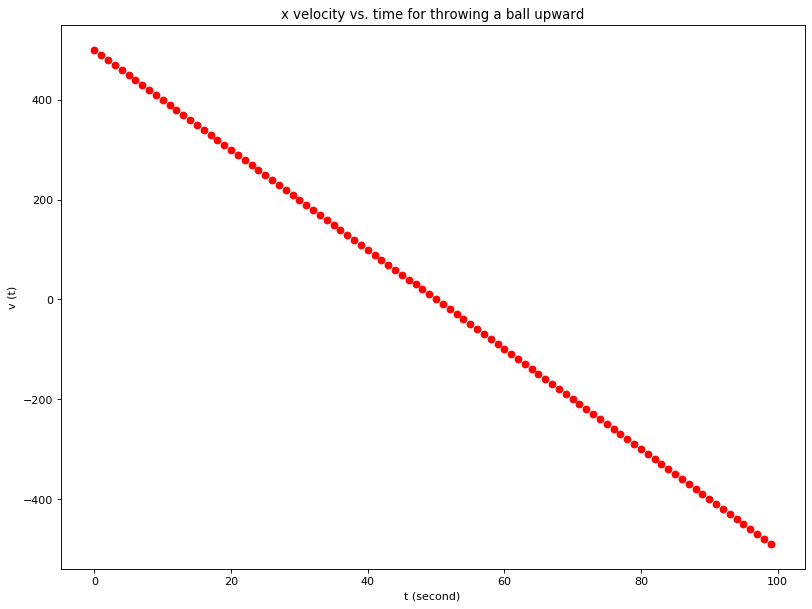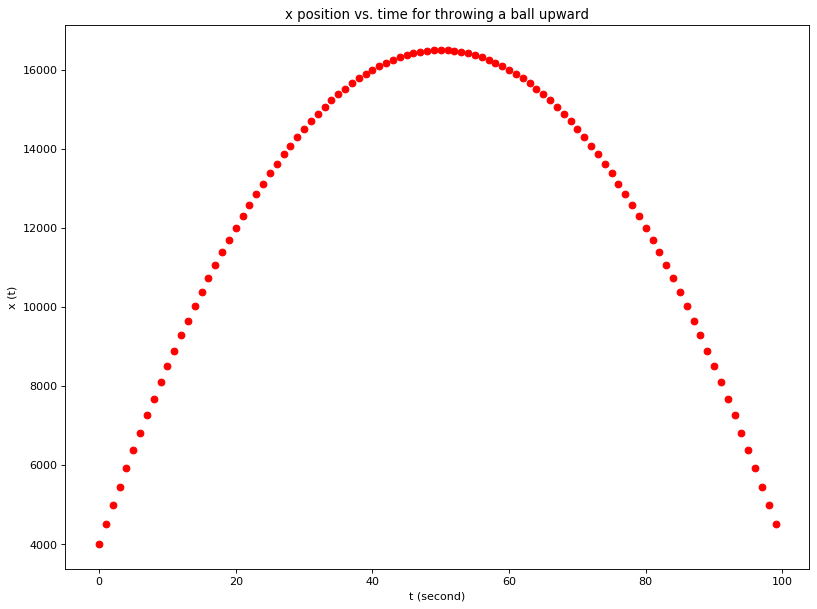One Dimensional MotionBelow is the one dimensional kinematic equation that we learn in physics class.

Given the initial position x1, initial speed v1 and acceleration a, the x2 position at time t can be calculated using the following formula.

$v_{2} = v_{1} + a \cdot \Delta {t}$ $x_{2} = x_{1} + v_{1} \cdot \Delta {t} + \frac{1}{2} \cdot a \cdot (\Delta t)^2$

Now we can use Python to calculate and visually see the result. These are the starting values:

• t = 100 seconds
• x1 = 4000 meters
• v1 = 500 m/s
• a = -10 m/s^2